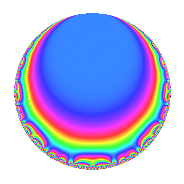# Properties

 Label 8008.2.a.fLevel 8008 Weight 2 Character orbit 8008.a Self dual Yes Analytic conductor 63.944 Analytic rank 0 Dimension 2 CM No Inner twists 1

# Related objects

## Newspace parameters

 Level: $$N$$ = $$8008 = 2^{3} \cdot 7 \cdot 11 \cdot 13$$ Weight: $$k$$ = $$2$$ Character orbit: $$[\chi]$$ = 8008.a (trivial)

## Newform invariants

 Self dual: Yes Analytic conductor: $$63.9442019386$$ Analytic rank: $$0$$ Dimension: $$2$$ Coefficient field: $$\Q(\sqrt{5})$$ Coefficient ring: $$\Z[a_1, a_2, a_3]$$ Coefficient ring index: $$1$$ Fricke sign: $$-1$$ Sato-Tate group: $\mathrm{SU}(2)$

## $q$-expansion

Coefficients of the $$q$$-expansion are expressed in terms of $$\beta = \frac{1}{2}(1 + \sqrt{5})$$. We also show the integral $$q$$-expansion of the trace form.

 $$f(q)$$ $$=$$ $$q + \beta q^{3} + ( 1 - \beta ) q^{5} - q^{7} + ( -2 + \beta ) q^{9} +O(q^{10})$$ $$q + \beta q^{3} + ( 1 - \beta ) q^{5} - q^{7} + ( -2 + \beta ) q^{9} + q^{11} - q^{13} - q^{15} + ( -4 + 3 \beta ) q^{17} + ( -1 + 5 \beta ) q^{19} -\beta q^{21} + ( -4 + 4 \beta ) q^{23} + ( -3 - \beta ) q^{25} + ( 1 - 4 \beta ) q^{27} + \beta q^{33} + ( -1 + \beta ) q^{35} + ( 6 - 2 \beta ) q^{37} -\beta q^{39} + ( -2 - 6 \beta ) q^{41} + ( -4 + 5 \beta ) q^{43} + ( -3 + 2 \beta ) q^{45} -4 q^{47} + q^{49} + ( 3 - \beta ) q^{51} + ( 6 + \beta ) q^{53} + ( 1 - \beta ) q^{55} + ( 5 + 4 \beta ) q^{57} + 6 q^{59} + ( 6 + 3 \beta ) q^{61} + ( 2 - \beta ) q^{63} + ( -1 + \beta ) q^{65} + ( 10 + \beta ) q^{67} + 4 q^{69} + ( 5 + 5 \beta ) q^{71} + ( 4 - 6 \beta ) q^{73} + ( -1 - 4 \beta ) q^{75} - q^{77} + ( 3 + \beta ) q^{79} + ( 2 - 6 \beta ) q^{81} + ( 11 - 3 \beta ) q^{83} + ( -7 + 4 \beta ) q^{85} + ( -6 + 3 \beta ) q^{89} + q^{91} + ( -6 + \beta ) q^{95} + ( -2 - 8 \beta ) q^{97} + ( -2 + \beta ) q^{99} +O(q^{100})$$ $$\operatorname{Tr}(f)(q)$$ $$=$$ $$2q + q^{3} + q^{5} - 2q^{7} - 3q^{9} + O(q^{10})$$ $$2q + q^{3} + q^{5} - 2q^{7} - 3q^{9} + 2q^{11} - 2q^{13} - 2q^{15} - 5q^{17} + 3q^{19} - q^{21} - 4q^{23} - 7q^{25} - 2q^{27} + q^{33} - q^{35} + 10q^{37} - q^{39} - 10q^{41} - 3q^{43} - 4q^{45} - 8q^{47} + 2q^{49} + 5q^{51} + 13q^{53} + q^{55} + 14q^{57} + 12q^{59} + 15q^{61} + 3q^{63} - q^{65} + 21q^{67} + 8q^{69} + 15q^{71} + 2q^{73} - 6q^{75} - 2q^{77} + 7q^{79} - 2q^{81} + 19q^{83} - 10q^{85} - 9q^{89} + 2q^{91} - 11q^{95} - 12q^{97} - 3q^{99} + O(q^{100})$$

## Embeddings

For each embedding $$\iota_m$$ of the coefficient field, the values $$\iota_m(a_n)$$ are shown below.

For more information on an embedded modular form you can click on its label.

Label $$\iota_m(\nu)$$ $$a_{2}$$ $$a_{3}$$ $$a_{4}$$ $$a_{5}$$ $$a_{6}$$ $$a_{7}$$ $$a_{8}$$ $$a_{9}$$ $$a_{10}$$
1.1
 −0.618034 1.61803
0 −0.618034 0 1.61803 0 −1.00000 0 −2.61803 0
1.2 0 1.61803 0 −0.618034 0 −1.00000 0 −0.381966 0
 $$n$$: e.g. 2-40 or 990-1000 Significant digits: Format: Complex embeddings Normalized embeddings Satake parameters Satake angles

## Inner twists

This newform does not admit any (nontrivial) inner twists.

## Atkin-Lehner signs

$$p$$ Sign
$$2$$ $$1$$
$$7$$ $$1$$
$$11$$ $$-1$$
$$13$$ $$1$$

## Hecke kernels

This newform can be constructed as the intersection of the kernels of the following linear operators acting on $$S_{2}^{\mathrm{new}}(\Gamma_0(8008))$$:

 $$T_{3}^{2} - T_{3} - 1$$ $$T_{5}^{2} - T_{5} - 1$$ $$T_{17}^{2} + 5 T_{17} - 5$$